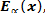Archive
Special IssuesVolume 2, Issue 1, March 2017, Page: 1-5
The Classical Laplace Transform and Its q-Image of the Most Generalized Hypergeometric and Mittag-Leffler Functions
D. K. Jain, Madhav Institute of Technology and Science, Gwalior (M.P.), India
Altaf Ahmad, School of Mathematics and Allied Sciences Jiwaji University, Gwalior (M.P.), India
Renu Jain, School of Mathematics and Allied Sciences Jiwaji University, Gwalior (M.P.), India
Farooq Ahmad, Department of Mathematics, Govt. Degree College, Kupwara (J&K), India
Received: Dec. 23, 2016;       Accepted: Jan. 16, 2017;       Published: Feb. 9, 2017
Abstract
The q-Calculus has served as a bridge between mathematics and physics, particularly in case of quantum physics. The q-generalizations of mathematical concepts like Laplace and Fourier transforms, Hypergeometric functions etc. can be advantageously used in solution of various problems arising in the field of physical and engineering sciences. The present paper deals with some of the important results of q-Laplace transform of Fox-Wright and Mittag-Leffler functions in terms of well-known Fox’s H-function. Some special cases have also been discussed.
Keywords
Classical Laplace Transform, q-Image of Laplace Transform, ML-Function, Fox-Wright Function
D. K. Jain, Altaf Ahmad, Renu Jain, Farooq Ahmad, The Classical Laplace Transform and Its q-Image of the Most Generalized Hypergeometric and Mittag-Leffler Functions, International Journal of Discrete Mathematics. Vol. 2, No. 1, 2017, pp. 1-5. doi: 10.11648/j.dmath.20170201.11
Reference

Sneddon, I. N. (1995). Fourier transforms. Courier Corporation.

Zemanian, A. H. (1968). Generalized integral transform, Pure Appl. Math. 18, inter Science Publ. John Wiley and Sons, New York.

Rao, G. L. N. (1974). The Generalized Laplace transform of generalized function, Ranchi Univ, Math. J.5.

Saxena, R. K. (1960). Some theorems on generalized Laplace transform I, Proc. Nat. Inst. Sci. India, part A, 26,400-413.

Mathai, A. M. (2007). A Versatile integral, Preprint series, No.12, Centre for Mathematical Sciences, Pala, Kerala, India.

Hahn, W. (1949). Beitrage Zur Theorie der Heineshan Reihen. Die 24 integrale der hypergeometrischen q-Differenzengleichung. Das q-Analogon der Laplace-Transformation. Mathematische Nachrichten, 2(6), 340-379.

Rooney, P. G. (1982\83). On integral transformation with G-function kernels, proc. Royal. Soc. Edinburgh Sect. A. 93.

Saxena, R. K. (1961). Some theorems on generalized Laplace transform II, Riv. Mat. Univ. Parma(2)2, 287-299.

Saxena, R. K. (1966). Some theorems on generalize Laplace transform, Proc. Cambridge Philos. Soc; 62, 467-471.

Purohit, S. D., Yadav R. K. and Vyas, V. K. On applications of q-Laplace transforms to a basic analogue of the I-function. (Communicated).

Purohit S. D., and Yadav, R. K. On q-Laplace transforms of certain q-hypergeometric polynomials , Proc. Nat. Acad. Sci. India(2006) 235-242-III.

Heideman, M. T., Johnson, D. H., & Burrus, C. S. (1985). Gauss and the history of the fast Fourier transform. Archive for history of exact sciences, 34(3), 265-277.

Kac, V., & Cheung, P. (2001). Quantum calculus. Springer Science & Business Media.

Wright, E. M (1935), The asymptotic expansion of generalized hypergeometric function. J. London Math. So, 10, 286-293.

Mittag-Leffler function, M. G.: 1903 Sur la nouvelle function, Comptes Rendus Acad. Sci, Paris (Ser.II)137, 554-558.

Metzler, R and Klafter, J.: The random walk’s guide to anomalous diffusion: a fractional dynamics approach, phys. Rep. 339(2000), 1-77.

Prabhakar, T. R (1971), A singular integral equation with a generalized Mittag-Leffler function in the kernel, Yokohama Math. J. Vol. 19, 7-15.

Sheikh, F. A., & Jain, D. K. Basic Hypergeometric Functions and q-Calculus. Corona publications (2015).

Sharma, S. K., & Jain, R. On Some Properties Of Generalized q-Mittag-Leffler Function. Mathematica Aeterna, Vol. 4, 2014, no.6, 613–619.